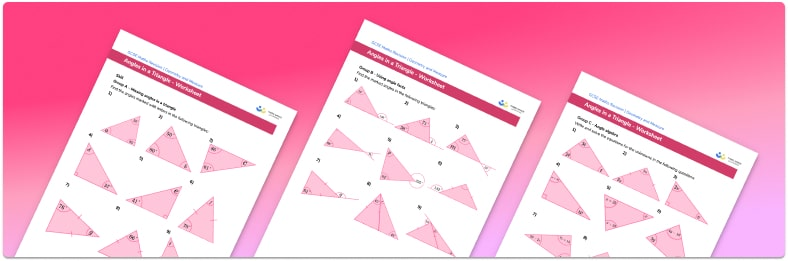# Angles In A Triangle Worksheet• Section 1 of the angles in a triangle worksheet contains 27 skills-based angles in a triangle questions, in 3 groups to support differentiation
• Section 2 contains 3 applied angles in a triangle questions with a mix of word problems and deeper problem solving questions
• Section 3 contains 3 foundation and higher level GCSE exam style questions
• Answers and a mark scheme for all questions are provided
• Questions follow variation theory with plenty of opportunities for students to work independently at their own level
• All questions created by fully qualified expert secondary maths teachers
• Suitable for GCSE maths revision for AQA, OCR and Edexcel exam boards

• This field is for validation purposes and should be left unchanged.

You can unsubscribe at any time (each email we send will contain an easy way to unsubscribe). To find out more about how we use your data, see our privacy policy.

### Angles in a triangle at a glance

Triangles can be classified based on their angles. An equilateral triangle is a regular polygon and has three equal angles. An isosceles triangle has two equal angles and two equal sides, and a scalene triangle has no equal angles and no equal sides. However, for all of these triangles, the angles of a triangle sum to 180°. This angle fact is useful for solving problems which require students to find missing angles in triangles. Angle problems don’t usually contain difficult fractions, but answers often need to be rounded to a given degree of accuracy.

So for triangle xyz, with angles y and z known, to find the size of angle x, we would work out y+z and subtract the result from 180°.

If the type of triangle is specified, such as an isosceles triangle, we can work out the size of two missing angles given the size of the third. If we know the size of one base angle in an isosceles triangle, we can use the fact that base angles in an isosceles triangle are equal in order to find the size of the other base angle. If we subtract the sum of the two angles from 180°, this gives us the size of the third angle.

It is important that students recognise a right angle when it is marked with the ∟ symbol rather than the value 90°.

The sum of the angles of a triangle can be extended further to applications involving algebraic expressions and solving equations.

Looking forward, students can then progress to additional angles in polygons worksheets and other geometry worksheets, for example an angles in polygons worksheet or area of a triangle worksheet.For more teaching and learning support on Geometry our GCSE maths lessons provide step by step support for all GCSE maths concepts.

## Do you have KS4 students who need more focused attention to succeed at GCSE?There will be students in your class who require individual attention to help them succeed in their maths GCSEs. In a class of 30, it’s not always easy to provide.

Help your students feel confident with exam-style questions and the strategies they’ll need to answer them correctly with our dedicated GCSE maths revision programme.

Lessons are selected to provide support where each student needs it most, and specially-trained GCSE maths tutors adapt the pitch and pace of each lesson. This ensures a personalised revision programme that raises grades and boosts confidence.

Find out more